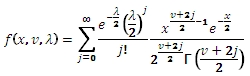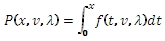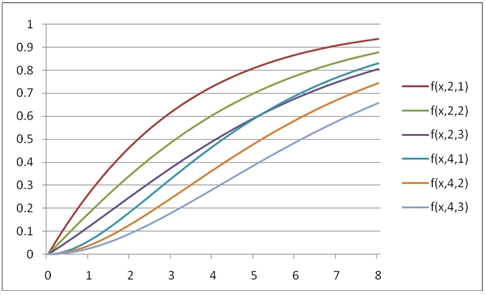# SQL Server non-central chi-squared distribution function

CHI2NC

Updated: 31 July 2010

Use CHI2NC to calculate the lower cumulative distribution function of the non-central chi-square distribution.

The probability density function is:The lower cumulative distribution function is:Syntax
SELECT [wctStatistics].[wct].[CHI2NC] (
<@X, float,>
,<@DF, float,>
,<@Lambda, float,>)
Arguments
@X
is any real number. @X is an expression of type float or of a type that implicitly converts to float.
@DF
is the degrees of freedom. @DF is an expression of type float or of a type that implicitly converts to float.
@Lambda
is the non-centrality parameter. @Lambda is an expression of type float or of a type that implicitly converts to float.
Return Types
float
Remarks
·         @DF must be greater than zero (@DF > 0).
·         @Lambda must be greater than or equal to zero (@Lambda ≥ 0).
·         @X must be greater than or equal to zero (@X ≥ zero).
Examples
SELECT wct.CHI2NC(1,3,3)
This produces the following result
----------------------
0.0582469145317944

(1 row(s) affected)

You can use the SeriesFloat function from the XLeratorDB/math library to generate a dataset which can be pasted into EXCEL to generate a graph of the function.
SELECT SeriesValue
,wct.CHI2NC(SeriesValue, 2, 1) as [f(x,2,1)]
,wct.CHI2NC(SeriesValue, 2, 2) as [f(x,2,2)]
,wct.CHI2NC(SeriesValue, 2, 3) as [f(x,2,3)]
,wct.CHI2NC(SeriesValue, 4, 1) as [f(x,4,1)]
,wct.CHI2NC(SeriesValue, 4, 2) as [f(x,4,2)]
,wct.CHI2NC(SeriesValue, 4, 3) as [f(x,4,3)]
FROM wct.SeriesFloat(0,8,.1,NULL,NULL)

This is an EXCEL-generated graph of the results### SupportCopyright 2008-2021 Westclintech LLC         Privacy Policy        Terms of Service